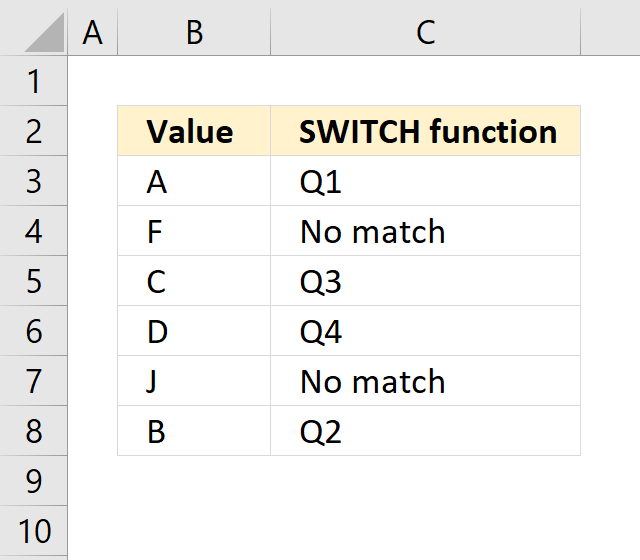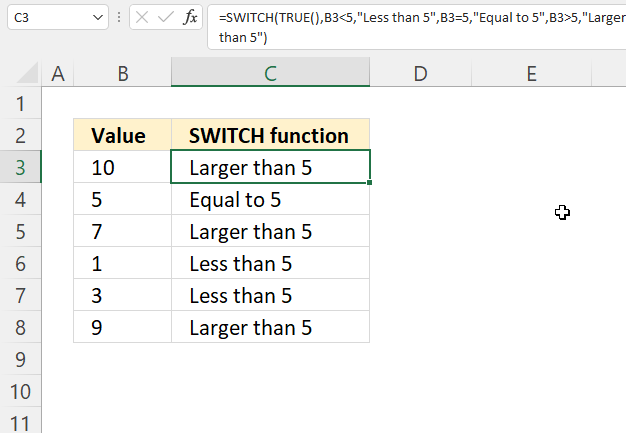Author: Oscar Cronquist Article last updated on May 30, 2022The SWITCH function returns a given value determined by an expression and a list of values. If the expression matches one of the values in the list the corresponding result value is returned. If no value matches an optional default value is returned by the function.

## 1. SWITCH Function Syntax

SWITCH(expression, value1, result1, [default or value2, result2],…[default or value3, result3])

## 2. SWITCH Function Arguments

 expression Required. This argument determines which value to be returned. If it matches the value argument the corresponding value in the result argument is returned. value1 Required. If this value matches the expression the corresponding result argument is returned. result1 Required. The value to be returned. [value2] Optional. Up to 125 additional arguments. [result2] Optional. Up to 125 additional arguments. [default] Optional. This is the value to be returned if no other value matches, this must be the last argument in the function.

This feature is only available for Excel 2019 and later Excel versions.

## 3. SWITCH Function exampleThis example demonstrates how to use the SWITCH function. The first argument changes when you copy the formula to cells below, ther remaining arguments are "hardcoded" meaning they don't change.

Formula in cell D3:

=SWITCH(C3,"A","Q1","B","Q2","C","Q3","D","Q4","No match")

The last argument lets you specify a value that is returned if no other value is matching.

## 4. SWITCH function - how to use logical operators like larger than and smaller thanThe SWITCH function is made for exact matches, however, there is a workaround to use larger than and smaller than characters.

The value1 argument lets you also create a test expression, if it matches the expression argument the result1 argument is returned. The following formula demonstrates this technique.

Keep in mind that the order of arguments is important in some cases.

Formula in cell C3:

=SWITCH(TRUE,B3<5,"Less than 5",B3=5,"Equal to 5",B3>5,"Larger than 5")

This example covers every possible number, the last argument is not necessary.

### Explaining formula

SWITCH(expression, value1, result1, [default or value2, result2],…[default or value3, result3])

#### Step 1 - Expression argument in cell C3

If any of the value arguments match the expression argument the corresponding result argument is returned.

TRUE is a boolean value that is often used in Excel formula, every logical test returns TRUE or FALSE or their numerical equivalents.

TRUE - 1
FALSE - 0 (zero)

#### Step 2 - First logical test (value1 argument)

The less than character lets you check if a number is smaller than another number, the result is a boolean value TRUE or FALSE.

B3<5

becomes

10<5

and returns FALSE. 10 is not smaller than 5.

#### Step 3 - Second logical test (value2 argument)

The equal sign lets you compare value to value, the result is either TRUE of FALSE, this is not a case sensitive comparison. Use the EXACT function for case-sensitive comparisons.

B3=5

becomes

10 = 5

and returns FALSE. 10 is not equal to 5.

#### Step 4 - Third logical test (value3 argument)

The larger than character is also a logical operator, the result is TRUE or FALSE like the other two described above.

B3>5

becomes

10>5

and returns TRUE. TRUE matches the first argument expression, the formula will now evaluate the corresponding result argument.

#### Step 5 - Third result3 argument

SWITCH(TRUE,B3<5,"Less than 5",B3=5,"Equal to 5",B3>5,"Larger than 5")

becomes

SWITCH(TRUE, FALSE, "Less than 5", FALSE, "Equal to 5", TRUE, "Larger than 5")

and returns "Larger than 5" in cell C3.